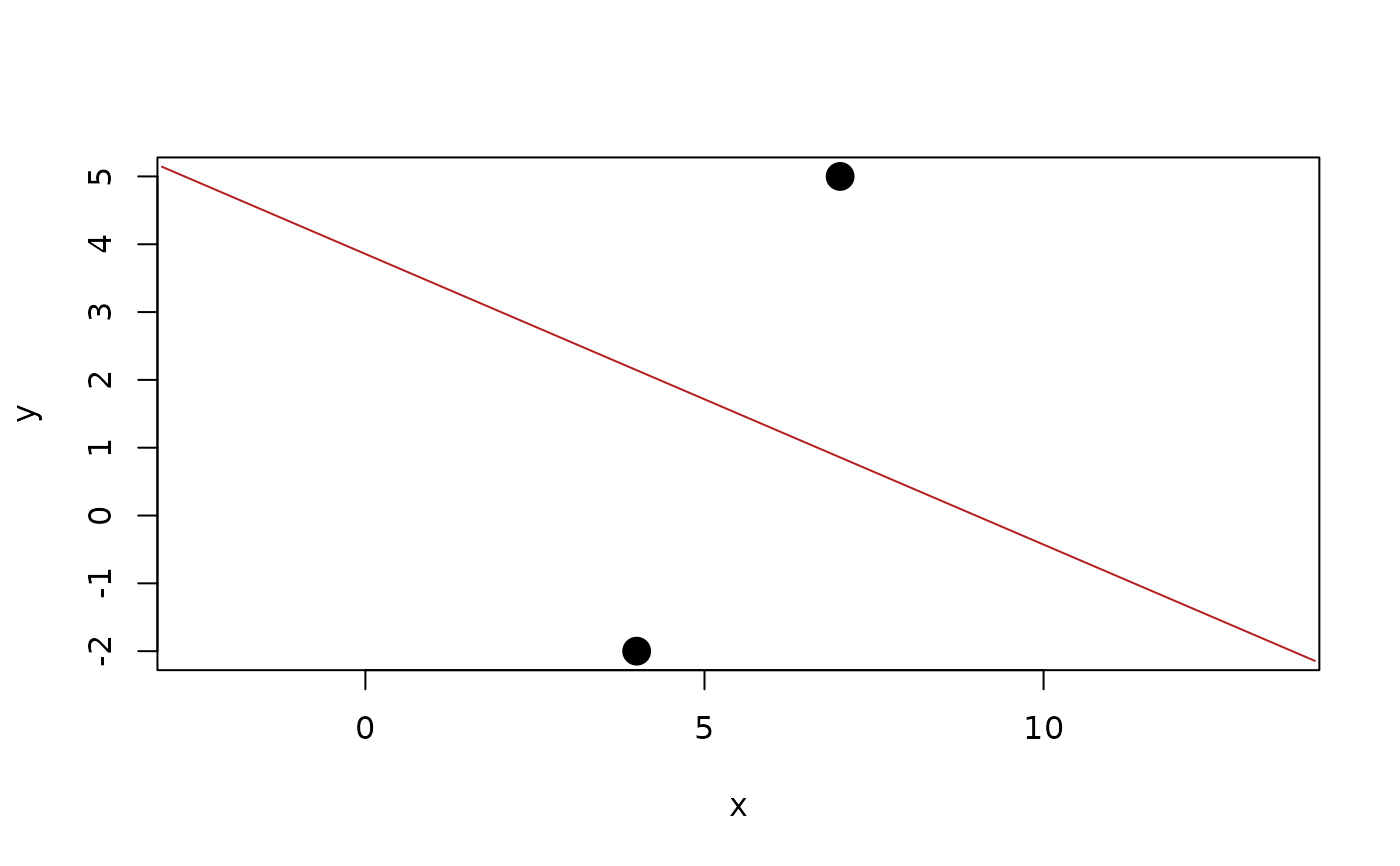A bisector is a geometry that splits the space in two, i.e. a line in 2D and a plane in 3D. Constructing a bisector from 2 geometries creates a split where the edge at any given point has equal length to the two geometries.

bisector(x, y)

x, y

Vector of points

## Value

A vector of lines or planes depending on the dimensionality of x and y

Other Locations: barycenter(), centroid(), circumcenter(), equidistant_line(), point(), radical(), weighted_point()

## Examples

b <- bisector(point(4, -2), point(7, 5))
b
#> <2D lines >
#>  <a:-6, b:-14, c:54>

plot(c(point(4, -2), point(7, 5)), pch = 16, cex = 2)
euclid_plot(b, col = "firebrick")